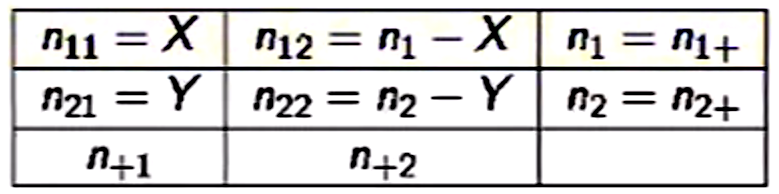### RELATIVE RISK AND ODDS RATIO:RELATIVE RISK:

It is defined as one proportion divided by another. For instance, the proportion of people developing a disease exposed to an environmental factor over the proportion of people who develop the disease but are not exposed to it.

The natural estimator of the population RR is the measured RR:

$$\huge RR= \frac{\frac{Prop.\,Disease}{Exposure}}{\frac{Prop\,Disease}{No\,Exposure}} =\,\frac{p_1}{p_2} = \frac{\frac{X}{n_1}}{\frac{Y}{n_2}}$$

To avoid the possibility of a zero in the denominator (counts are zero bounded) we take the natural $$log$$ of the RR, and use it to calculate confidence intervals. The standard error will be:

$$\Large SE_{logRR} =\Bigg(\frac{(1-p_1)}{p_1n_1}\,+\,\frac{(1-p_2)}{p_2n_2}\Bigg)^{1/2}$$

The CI of the log_RR is:

$$RR \pm qnorm(0.975) SE_{logRR}$$.

To get the CI of the RR just exponentiate the endpoints.

ODDS RATIO:

$$\huge OR=\frac{\frac{Odds\,Disease}{Exposure}}{\frac{Odds\,Disease}{No\,Exposure}}=\frac{p_1/(1-p_1)}{p_2/(1-p_2)}=\frac{p_1(1-p_2)}{p_2(1-p_1)}$$

The sample odds ratio simply turns out to be:

$$\huge \frac{p_1/(1-p_1)}{p_2/(1-p_2)}=\frac{n_{11}\,n_{22}}{n_{12}\,n_{21}}$$

The SE of the log of the OR is:

$$\Large SE_{logOR}=\sqrt{\frac{1}{n_{11}}+\frac{1}{n_{12}}+\frac{1}{n_{21}}+\frac{1}{n_{22}}}$$

The CI of the log_OR is:

$$OR \pm qnorm(0.975) SE_{logOR}$$.

And we exponentiate the ends.